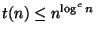Next: NPO problems: definitions and Up: A compendium of NP Previous: A compendium of NP   Index

Introduction

Due to the fact that no NP-complete problem can be solved in polynomial time (unless P=NP), many approximability results (both positive and negative) of NP-hard optimization problems have appeared in the technical literature. In this compendium, we collect a large number of these results.

In the following we refer to standard complexity classes (see ). We recall that a function t(n) is `quasi-polynomial' if a constant c exists such thatand we denote by QP, QNP, and QR the analogues of the usual complexity classes in the quasi-polynomial time domain.

Viggo Kann
2000-03-20